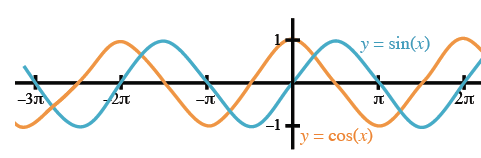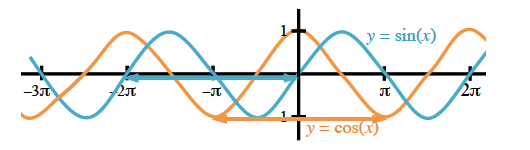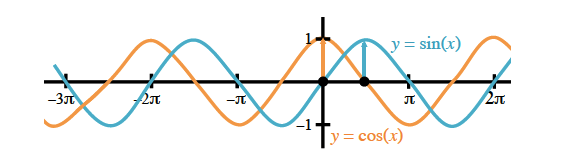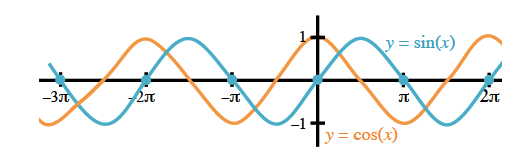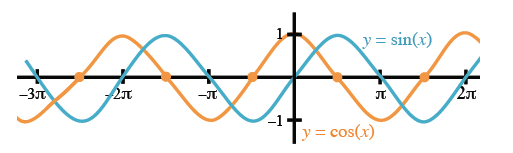### Home > PC > Chapter 4 > Lesson 4.1.3 > Problem4-38

4-38.
1. ARE SINE AND COSINE PARENT GRAPHS? Homework Help ✎

Although sine and cosine have the shape and one can be transformed by a shift to create the other, we will consider them both parent graphs. We have determined the domain and range for both functions. Complete the investigation by answering the following questions:

1. What is the period for y = sin x and y = cos x?

2. What is the amplitude for y = sin x and y = cos x?

3. What are the intercepts for y = sin x?

4. What are the intercepts for y = cos x?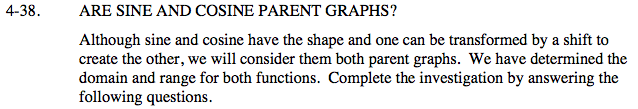Use the graphs below to find the answers for (a) - (d).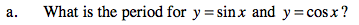The x distance for 1 whole cycle.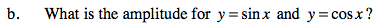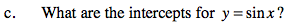Write an expression for all intercepts.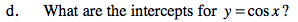Write an expression for all intercepts.